# Points, lines, and planes

In what follows are various notes and algorithms dealing with points, lines, and planes.

# Minimum Distance between a Point and a Line

Written by Paul Bourke
October 1988

This note describes the technique and gives the solution to finding the shortest distance from a point to a line or line segment. The equation of a line defined through two points P1 (x1,y1) and P2 (x2,y2) is

P = P1 + u (P2 - P1)The point P3 (x3,y3) is closest to the line at the tangent to the line which passes through P3, that is, the dot product of the tangent and line is 0, thus

(P3 - P) dot (P2 - P1) = 0

Substituting the equation of the line gives

[P3 - P1 - u(P2 - P1)] dot (P2 - P1) = 0

Solving this gives the value of u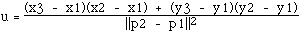Substituting this into the equation of the line gives the point of intersection (x,y) of the tangent as

x = x1 + u (x2 - x1)
y = y1 + u (y2 - y1)

The distance therefore between the point P3 and the line is the distance between (x,y) above and P3.

Notes

• The only special testing for a software implementation is to ensure that P1 and P2 are not coincident (denominator in the equation for u is 0)

• If the distance of the point to a line segment is required then it is only necessary to test that u lies between 0 and 1.

• The solution is similar in higher dimensions.

Source code contributions
C source from Damian Coventry: C source code
VBA from Brandon Crosby: VBA source code
Dephi from Graham O'Brien: Delphi version
"R" version from Gregoire Thomas: pointline.r
JAVA version from Pieter Iserbyt: DistancePoint.java
LabView implementation from Chris Dancer: Pointlinesegment.vi.zip
Right side distance by Orion Elenzil: rightside
VBA VB6 by Thomas Ludewig: vbavb6.txt

# Minimum Distance between a Point and a Plane

Written by Paul Bourke
March 1996

Let Pa = (xa, ya, za) be the point in question.

A plane can be defined by its normal n = (A, B, C) and any point on the plane Pb = (xb, yb, zb)

Any point P = (x,y,z) lies on the plane if it satisfies the following

A x + B y + C z + D = 0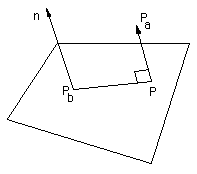The minimum distance between Pa and the plane is given by the absolute value of

 (A xa + B ya + C za + D) / sqrt(A2 + B2 + C2) . . . 1

To derive this result consider the projection of the line (Pa - Pb) onto the normal of the plane n, that is just ||Pa - Pb|| cos(theta), where theta is the angle between (Pa - Pb) and the normal n. This projection is the minimum distance of Pa to the plane.

This can be written in terms of the dot product as

minimum distance = (Pa - Pb) dot n / ||n||

That is

 minimum distance = (A (xa - xb) + B (ya - yb) + C (za - zb)) / sqrt(A2 + B2 + C2) . . . 2

Since point (xb, yb, zb) is a point on the plane

 A xb + B yb + C zb + D = 0 . . . 3

Substituting equation 3 into equation 2 gives the result shown in equation 1.

# Intersection point of two line segments in 2 dimensions

Written by Paul Bourke
April 1989

This note describes the technique and algorithm for determining the intersection point of two lines (or line segments) in 2 dimensions.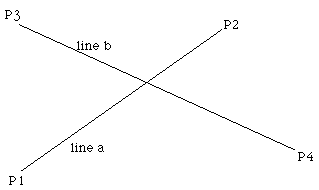The equations of the lines are

Pa = P1 + ua ( P2 - P1 )

Pb = P3 + ub ( P4 - P3 )

Solving for the point where Pa = Pb gives the following two equations in two unknowns (ua and ub)

x1 + ua (x2 - x1) = x3 + ub (x4 - x3)

and
y1 + ua (y2 - y1) = y3 + ub (y4 - y3)

Solving gives the following expressions for ua and ub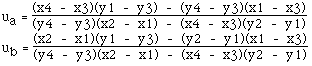Substituting either of these into the corresponding equation for the line gives the intersection point. For example the intersection point (x,y) is

x = x1 + ua (x2 - x1)

y = y1 + ua (y2 - y1)

Notes:

• The denominators for the equations for ua and ub are the same.

• If the denominator for the equations for ua and ub is 0 then the two lines are parallel.

• If the denominator and numerator for the equations for ua and ub are 0 then the two lines are coincident.

• The equations apply to lines, if the intersection of line segments is required then it is only necessary to test if ua and ub lie between 0 and 1. Whichever one lies within that range then the corresponding line segment contains the intersection point. If both lie within the range of 0 to 1 then the intersection point is within both line segments.

Source code contributions
Original C code by Paul Bourke.
Python version by Yuangan Zhou.
C++ contribution by Damian Coventry.
LISP implementation by Paul Reiners.
C version for Rockbox firmware by Karl Kurbjun.
C# version by Olaf Rabbachin.
VB.net version by Olaf Rabbachin.
VBA implementation by Giuseppe Iaria.
Javascript version by Leo Bottaro.

# The shortest line between two lines in 3D

Written by Paul Bourke
April 1998

Two lines in 3 dimensions generally don't intersect at a point, they may be parallel (no intersections) or they may be coincident (infinite intersections) but most often only their projection onto a plane intersect.. When they don't exactly intersect at a point they can be connected by a line segment, the shortest line segment is unique and is often considered to be their intersection in 3D.

 The following will show how to compute this shortest line segment that joins two lines in 3D, it will as a bi-product identify parallel lines. In what follows a line will be defined by two points lying on it, a point on line "a" defined by points P1 and P2 has an equation. Pa = P1 + mua (P2 - P1) similarly a point on a second line "b" defined by points P4 and P4 will be written as Pb = P3 + mub (P4 - P3) The values of mua and mub range from negative to positive infinity. The line segments between P1 P2 and P3 P4 have their corresponding mu between 0 and 1.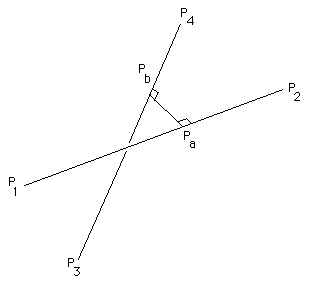There are two approaches to finding the shortest line segment between lines "a" and "b". The first is to write down the length of the line segment joining the two lines and then find the minimum. That is, minimise the following

|| Pb - Pa ||2

Substituting the equations of the lines gives

|| P1 - P3 + mua (P2 - P1) - mub (P4 - P3) ||2

The above can then be expanded out in the (x,y,z) components. There are conditions to be met at the minimum, the derivative with respect to mua and mub must be zero. Note: it is easy to convince oneself that the above function only has one minima and no other minima or maxima. These two equations can then be solved for mua and mub, the actual intersection points found by substituting the values of mu into the original equations of the line.

An alternative approach but one that gives the exact same equations is to realise that the shortest line segment between the two lines will be perpendicular to the two lines. This allows us to write two equations for the dot product as

(Pa - Pb) dot (P2 - P1) = 0

(Pa - Pb) dot (P4 - P3) = 0

Expanding these given the equation of the lines

( P1 - P3 + mua (P2 - P1) - mub (P4 - P3) ) dot (P2 - P1) = 0

( P1 - P3 + mua (P2 - P1) - mub (P4 - P3) ) dot (P4 - P3) = 0

Expanding these in terms of the coordinates (x,y,z) is a nightmare but the result is as follows

d1321 + mua d2121 - mub d4321 = 0

d1343 + mua d4321 - mub d4343 = 0

where

dmnop = (xm - xn)(xo - xp) + (ym - yn)(yo - yp) + (zm - zn)(zo - zp)

Note that dmnop = dopmn

Finally, solving for mua gives

mua = ( d1343 d4321 - d1321 d4343 ) / ( d2121 d4343 - d4321 d4321 )

and back-substituting gives mub

mub = ( d1343 + mua d4321 ) / d4343

Source code contributions
Original C source code from the author: lineline.c
Contribution by Dan Wills in MEL (Maya Embedded Language): source.mel.
A Matlab version by Cristian Dima: linelineintersect.m.
A Maxscript function by Chris Johnson: LineLineIntersect.ms
LISP version for AutoCAD (and Intellicad) by Andrew Bennett: int1.lsp and int2.lsp
A contribution by Bruce Vaughan in the form of a Python script for the SDS/2 design software: L3D.py
C# version by Ronald Holthuizen: calclineline.cs
VBA VB6 version by Thomas Ludewig: vbavb6.txt
LabView implementation by John van Schaijk: V01_LineLine3D.vi.zip

# Intersection of a plane and a line

Written by Paul Bourke
August 1991

Contribution by Bryan Hanson: Implementation in R
and Graham Wise: C#

This note will illustrate the algorithm for finding the intersection of a line and a plane using two possible formulations for a plane.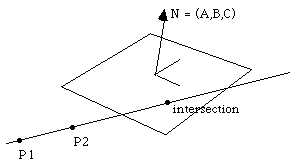### Solution 1

The equation of a plane (points P are on the plane with normal N and point P3 on the plane) can be written as

N dot (P - P3) = 0

The equation of the line (points P on the line passing through points P1 and P2) can be written as

P = P1 + u (P2 - P1)

The intersection of these two occurs when

N dot (P1 + u (P2 - P1)) = N dot P3

Solving for u gives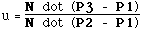Note

• If the denominator is 0 then the normal to the plane is perpendicular to the line. Thus the line is either parallel to the plane and there are no solutions or the line is on the plane in which case there are an infinite number of solutions

• If it is necessary to determine the intersection of the line segment between P1 and P2 then just check that u is between 0 and 1.

### Solution 2

A plane can also be represented by the equation

A x + B y + C z + D = 0

where all points (x,y,z) lie on the plane.

Substituting in the equation of the line through points P1 (x1,y1,z1) and P2 (x2,y2,z2)

P = P1 + u (P2 - P1)

gives

A (x1 + u (x2 - x1)) + B (y1 + u (y2 - y1)) + C (z1 + u (z2 - z1)) + D = 0

Solving for u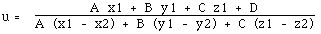Note

• the denominator is 0 then the normal to the plane is perpendicular to the line. Thus the line is either parallel to the plane and there are no solutions or the line is on the plane in which case are infinite solutions

• if it is necessary to determine the intersection of the line segment between P1 and P2 then just check that u is between 0 and 1.

# Equation of a plane

Written by Paul Bourke
March 1989
 The standard equation of a plane in 3 space is Ax + By + Cz + D = 0 The normal to the plane is the vector (A,B,C).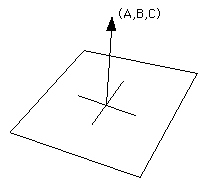Given three points in space (x1,y1,z1), (x2,y2,z2), (x3,y3,z3) the equation of the plane through these points is given by the following determinants.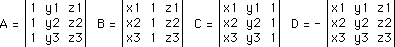Expanding the above gives
A = y1 (z2 - z3) + y2 (z3 - z1) + y3 (z1 - z2)
B = z1 (x2 - x3) + z2 (x3 - x1) + z3 (x1 - x2)
C = x1 (y2 - y3) + x2 (y3 - y1) + x3 (y1 - y2)
- D = x1 (y2 z3 - y3 z2) + x2 (y3 z1 - y1 z3) + x3 (y1 z2 - y2 z1)

Note that if the points are collinear then the normal (A,B,C) as calculated above will be (0,0,0).

The sign of s = Ax + By + Cz + D determines which side the point (x,y,z) lies with respect to the plane. If s > 0 then the point lies on the same side as the normal (A,B,C). If s < 0 then it lies on the opposite side, if s = 0 then the point (x,y,z) lies on the plane.

Alternatively

If vector N is the normal to the plane then all points p on the plane satisfy the following

N . p = k

where . is the dot product between the two vectors.

ie: a . b = (ax,ay,az) . (bx,by,bz) = ax bx + ay by + az bz

Given any point a on the plane

N . (p - a) = 0

# The intersection of two planes

Written by Paul Bourke
February 2000

The intersection of two planes (if they are not parallel) is a line.

Define the two planes with normals N as

N1 . p = d1

N2 . p = d2

The equation of the line can be written as

p = c1 N1 + c2 N2 + u N1 * N2

Where "*" is the cross product, "." is the dot product, and u is the parameter of the line.

Taking the dot product of the above with each normal gives two equations with unknowns c1 and c2.

N1 . p = d1 = c1 N1 . N1 + c2 N1 . N2

N2 . p = d2 = c1 N1 . N2 + c2 N2 . N2

Solving for c1 and c2

c1 = ( d1 N2 . N2 - d2 N1 . N2 ) / determinant

c2 = ( d2 N1 . N1 - d1 N1 . N2) / determinant

determinant = ( N1 . N1 ) ( N2 . N2 ) - ( N1 . N2 )2

Note that a test should first be performed to check that the planes aren't parallel or coincident (also parallel), this is most easily achieved by checking that the cross product of the two normals isn't zero. The planes are parallel if

N1 * N2 = 0

# Intersection of three planes

Written by Paul Bourke
October 2001

A contribution by Bruce Vaughan in the form of a Python script for the SDS/2 design software: P3D.py.

The intersection of three planes is either a point, a line, or there is no intersection (any two of the planes are parallel).

The three planes can be written as

N1 . p = d1

N2 . p = d2

N3 . p = d3

In the above and what follows, "." signifies the dot product and "*" is the cross product. The intersection point P is given by:

 d1 ( N2 * N3 ) + d2 ( N3 * N1 ) + d3 ( N1 * N2 ) P = ------------------------------------------------------------------------- N1 . ( N2 * N3 )

The denominator is zero if N2 * N3 = 0, in other words the planes are parallel. Or if N1 is a linear combination of N2 and N3.

# Equation of a line in polar coordinates

Written by Paul Bourke
March 2013

How might one represent a line in polar coordinates?
In Cartesian coordinates it can be represented as: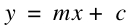The derivation is quite straightforward once one realises that for a point (r, theta) the x axis is simply r cos(theta) and the y axis is r sin(theta). Substituting those into the equation for the line gives the following result.Example from the Graphing Calculator.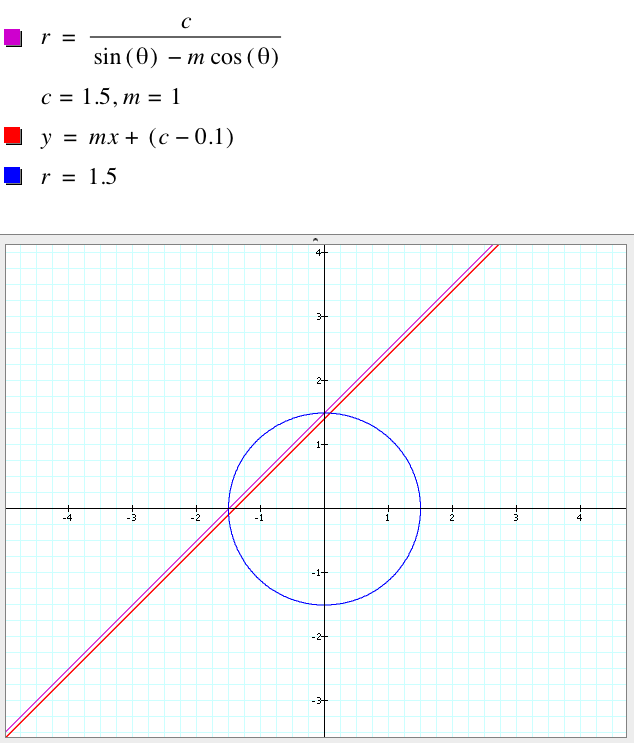Question

Given a line defined by two points L1 L2, a point P1 and angle z (bearing from north) find the intersection point between the direction vector from P1 to the line.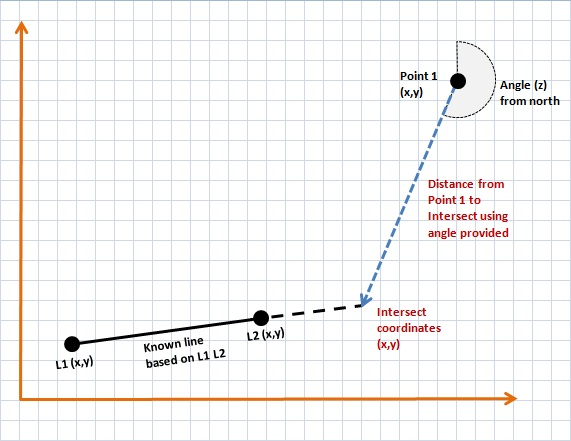Short answer: choose a second point P2 along the direction vector from P1, say P2 = (xP1+sin(z),yP1+cos(z)). Apply the algorthm here for the intersection of two line segments. Perform the additional test that ub must be greater than 0, the solution where ub is less than 0 is the solution in the direction z+180 degrees.